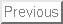Line Segments

Most computer programs represent geometry as arrangements of line segments. Arbitrary closed curves or shapes can be represented by ordered collections of line segments or polygons.

A line segment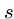is the portion of a line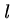which lies between two given points inclusive. Thus line segments are most naturally represented by pairs of endpoints:


typedef struct {
point p1,p2;            /* endpoints of line segment */
} segment;


The most important geometric primitive on segments, testing whether a given pair of them intersect, proves surprisingly complicated because of tricky special cases that arise.

The right way to deal with degeneracy is to base all computation on a small number of carefully crafted geometric primitives. Previously we implemented a general line data type that successfully dealt with vertical lines; those of infinite slope. We can reap the benefits by generalizing our line intersection routines to line segments.

Segment intersection can also be cleanly tested using a primitive to check whether three ordered points turn in a counterclockwise direction.

Testing Intersection


bool segments_intersect(segment s1, segment s2)
{
line l1,l2;      /* lines containing the input segments */
point p;         /* intersection point */

points_to_line(s1.p1,s1.p2,&l1);
points_to_line(s2.p1,s2.p2,&l2);

if (same_lineQ(l1,l2))  /* overlapping or disjoint segments */
return( point_in_box(s1.p1,s2.p1,s2.p2) ||
point_in_box(s1.p2,s2.p1,s2.p2) ||
point_in_box(s2.p1,s1.p1,s1.p2) ||
point_in_box(s2.p1,s1.p1,s1.p2) );

if (parallelQ(l1,l2)) return(FALSE);

intersection_point(l1,l2,p);

return(point_in_box(p,s1.p1,s1.p2) && point_in_box(p,s2.p1,s2.p2));
}



bool point_in_box(point p, point b1, point b2)
{
return( (p[X] >= min(b1[X],b2[X])) && (p[X] <= max(b1[X],b2[X]))
&& (p[Y] >= min(b1[Y],b2[Y])) && (p[Y] <= max(b1[Y],b2[Y])) );
}


We will use our line intersection routines to find an intersection point if one exists.

Polygons and Angle Computations

Polygons are closed chains of non-intersecting line segments. Instead of explicitly listing the segments (or edges) of polygon, we can implicitly represent them by listing thevertices in order around the boundary of the polygon. Thus a segment exists between the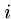th and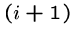st points in the chain for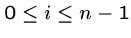. These indices are taken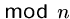to ensure there is an edge between the first and last point:


typedef struct {
int n;                  /* number of points in polygon */
point p[MAXPOLY];       /* array of points in polygon */
} polygon;


A polygon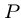is convex if any line segment defined by two points withinlies entirely within; i.e., there are no notches or bumps such that the segment can exit and re-enter. This implies that all internal angles in a convex polygon must be acute; i.e., at most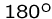or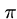radians.

Actually computing the angle defined between three ordered points is a tricky problem. We can avoid the need to know actual angles in most geometric algorithms by using the counterclockwise predicate ccw(a,b,c). This routine tests whether point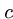lies to the right of the directed line which goes from point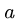to point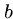.

Testing Angle Direction

These predicates are computed using signed_triangle_area(). Negative area results if pointis to the left of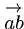. Zero area results if all three points are collinear.


bool ccw(point a, point b, point c)
{
double signed_triangle_area();

return (signed_triangle_area(a,b,c) > EPSILON);
}

bool cw(point a, point b, point c)
{
double signed_triangle_area();

return (signed_triangle_area(a,b,c) < EPSILON);
}

bool collinear(point a, point b, point c)
{
double signed_triangle_area();

return (fabs(signed_triangle_area(a,b,c)) <= EPSILON);
}


Convex Hulls

Convex hull is to computational geometry what sorting is to other algorithmic problems, a first step to apply to unstructured data so we can do more interesting things with it.

The convex hull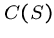of a set of points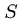is the smallest convex polygon containing.

The Graham's scan algorithm for convex hull which we will implement first sorts the points in angular order, and then incrementally inserts the points into the hull in this sorted order. Previous hull points rendered obsolete by the last insertion are then deleted.

Observe that both the leftmost and lowest points must lie on the hull, because they cannot lie within some other triangle of points.

The main loop of the algorithm inserts the points in increasing angular order around this initial point. Because of this ordering, the newly inserted point must sit on the hull of the thus-far-inserted points. This new insertion may form a triangle containing former hull points which now must be deleted. These points-to-be-deleted will sit at the end of the chain.

The deletion criteria is whether the new insertion makes an obtuse angle with the last two points on the chain. If the angle is too large, the last point on the chain has to go. We repeat until a small enough angle is created or we run out of points.


point first_point;              /* first hull point */

convex_hull(point in[], int n, polygon *hull)
{
int i;                  /* input counter */
int top;                /* current hull size */
bool smaller_angle();

if (n <= 3) {           /* all points on hull! */
for (i=0; i<n; i++)
copy_point(in[i],hull->p[i]);
hull->n = n;
return;
}

sort_and_remove_duplicates(in,&n);
copy_point(in,&first_point);

qsort(&in, n-1, sizeof(point), smaller_angle);

copy_point(first_point,hull->p);
copy_point(in,hull->p);

copy_point(first_point,in[n]);  /* sentinel for wrap-around */
top = 1;
i = 2;

while (i <= n) {
if (!ccw(hull->p[top-1], hull->p[top], in[i]))
top = top-1;    /* top not on hull */
else {
top = top+1;
copy_point(in[i],hull->p[top]);
i = i+1;
}
}

hull->n = top;
}


Avoiding Degeneracy

The beauty of this implementation is how naturally it avoids most of the problems of degeneracy.

A particularly insidious problem is when three or more input points are collinear. We resolve this by breaking ties in sorting by angle according to the distance from the initial hull point.


bool smaller_angle(point *p1, point *p2)
{
if (collinear(first_point,*p1,*p2)) {
if (distance(first_point,*p1) <= distance(first_point,*p2))
return(-1);
else
return(1);
}

if (ccw(first_point,*p1,*p2))
return(-1);
else
return(1);
}


The remaining degenerate case concerns repeated points. To eliminate this problem, we remove duplicate copies of points when we sort to identify the leftmost-lowest hull point:


sort_and_remove_duplicates(point in[], int *n)
{
int i;                  /* counter */
int oldn;               /* number of points before deletion */
int hole;               /* index marked for potential deletion */
bool leftlower();

qsort(in, *n, sizeof(point), leftlower);

oldn = *n;
hole = 1;
for (i=1; i<(oldn-1); i++) {
if ((in[hole-1][X]==in[i][X]) && (in[hole-1][Y]==in[i][Y]))
(*n)--;
else {
copy_point(in[i],in[hole]);
hole = hole + 1;
}
}
copy_point(in[oldn-1],in[hole]);
}

bool leftlower(point *p1, point *p2)
{
if ((*p1)[X] < (*p2)[X]) return (-1);
if ((*p1)[X] > (*p2)[X]) return (1);

if ((*p1)[Y] < (*p2)[Y]) return (-1);
if ((*p1)[Y] > (*p2)[Y]) return (1);

return(0);
}


There are a few final things to note about convex_hull. Observe the beautiful use of sentinels to simplify the code. Finally, note that we sort the points by angle without ever actually computing angles. The ccw predicate is enough to do the job.

Area of a Polygon

We can compute the area of any triangulated polygon by summing the area of all triangles. This is easy to implement using the routines developed in the text.

However, there is an even slicker algorithm based on the notion of signed areas for triangles, which we used as the basis for our ccw routine. By properly summing the signed areas of the triangles defined by an arbitrary pointwith each segment of polygonwe get the area of, because the negatively signed triangles cancel the area outside the polygon. This computation simplifies to the equation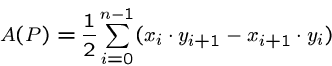where all indices are taken modulo the number of vertices.


double area(polygon *p)
{
double total = 0.0;             /* total area so far */
int i, j;                       /* counters */

for (i=0; i<p->n; i++) {
j = (i+1) % p->n;
total += (p->p[i][X]*p->p[j][Y]) - (p->p[j][X]*p->p[i][Y]);
}

return(total / 2.0);
}


Assigned Problems

111401 (Herding Frosh) - Fence in a set of points with the smallest amount of thread, including enough to tie the ends. What problem is this?

111403 (Chainsaw Massacre) - Find the largest empty rectangular area in a field of trees. Are the trees better represented by an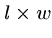matrix or in the compressed format of the input?

111404 (Hotter Colder) - Find the area of the region of possible locations for an object given certain hotter-colder'' constraints. How should we represent the region of possibilities? Is it always a convex polygon?

111408 (Nice Milk) - Maximize the wet area of a polygon with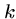dips into a depth-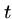milk dish? Does some form of greedy algorithm maximize wet area or must we use exhaustive search?